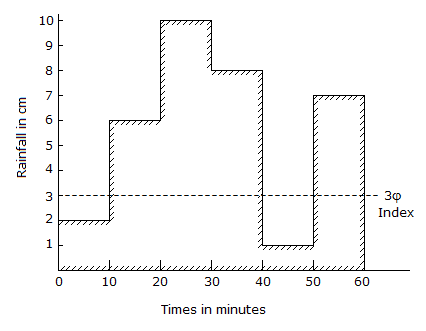# Civil Engineering - Water Resources Engineering - Discussion

### Discussion :: Water Resources Engineering - Section 2 (Q.No.27)

27.

The rate of rainfall for successive 10 minute periods of a 60 minute duration storm, are shown in the below figure. If the value of φindex is 3 cm/hour, the run off will be[A]. 2 cm [B]. 3 cm [C]. 4 cm [D]. 5 cm.

Explanation:

No answer description available for this question.

 Riajul said: (Dec 1, 2016) I think the answer should be 4.75cm.

 Mughal Saltanat said: (Dec 4, 2016) Answer should be 2.66 cm.

 Siva said: (Dec 5, 2016) (6 + 10 + 8 + 7) * 10/60 - (3 * 40/60) = 3.

 Kishan said: (Feb 7, 2017) Please, Give explanation with formula.

 Rajashekhar said: (Mar 30, 2017) You have to consider only graph above phi index it will be 19. Then runoff = (19 * 10)/60 = 3.1 approximately 3.

 Sikha said: (Oct 24, 2017) Can't understand. Can anyone provide some formula to solve this?

 Krunal said: (Mar 2, 2018) From the graph, the upper part of φindex is called runoff and lower part is called infiltration. Values are in min so we get convert its by divided by60 in hr. Upper part s values are from the graph are 6, 10, 8, 7 for 10 min period. R= (6-Πindex) *period/60 + (10-3) *10/60+ (8-3) *10/60+ (7-3) *10/60. So we can get the answer 3.

 Raaj said: (Aug 12, 2018) Thank you @Krunal, @Rajshekhar and @Shiva.

 Irfan said: (Jan 4, 2019) Thanks for the good explanation @Krunal.

 Yash said: (Jan 24, 2019) φindex=(P-R)/tr. where, tr= rainfall time (10 minutes). R= runoff. P= storm time (total time = 60minute). and φindex = 3 (given). By converting mm to cm we will get the answer is 3.

 Brijesh said: (Jul 6, 2019) I think 5 is Right.

 Appala Naidu said: (Jul 12, 2019) Here rainfall is given in cm but actually it is in mm. Then we can get an answer like.{(31-R) * 60}/40 = 3. By this, we can get R = 2.9 cm ~3cm.

 A G said: (Feb 9, 2020) \$ index = p-q/t. 3/60 = 60+70-q/60. q = 127. so, 60+70-127 = 3.